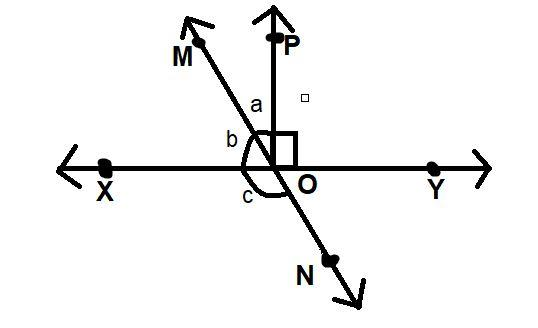QuestionAnswers

# In figure, lines XY and MN intersect at O. If $\angle POY = {90^0}$ and a: b=2:3, find c.Verified
113.7K+ Views
Hint: Here we go through by applying the properties of supplementary angle, complementary angles and then assume the given ratio with some variable as we let a=2x and b=3x. then apply the condition of angle to find the unknown angle.

Here in the given question the ratio of angle a: b is given as 2:3. So we have to let the $\angle POM = a = 2x$ and $\angle MOX = b = 3x$.
Given $\angle POY = {90^0}$ ,
So,$\angle POX$ will be ${180^0}$-$\angle POY = {90^0}$ (Because $XOY$ is straight line)
Then $\angle POX$=$\angle POM$+$\angle MOX$=${90^0}$
And as we know that $\angle POM$ and $\angle MOX$ are complementary because the sum of these two angles is ${90^0}$. I.e. $\angle POM + \angle MOX = {90^0}$ after putting the values of these angle we get,
$\Rightarrow 2x + 3x = {90^0} \\ \Rightarrow 5x = {90^0} \\ \therefore x = {18^0} \\$
$\angle POM = a = 2 \times {18^0} = {36^0}$ And $\angle MOX = b = 3 \times {18^0} = {54^0}$
And we also know that $\angle MOX$ and $\angle XON$ are supplementary angles because the sum of these two angles is ${180^0}$. i.e. $\angle MOX + \angle XON = {180^0}$
$\Rightarrow {54^0} + \angle XON = {180^0} \\ \Rightarrow \angle XON = {180^0} - {54^0} = {126^0} \\$
Hence the value of $\angle XON = c = {126^0}$.